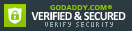ISBN: 1429240326

ISBN13: 9781429240321

# Introduction to the Practice of Statistics: W/Student CD [With CDROM]

## Recommended

Format: Hardcover

Condition: Acceptable

\$6.39
Save \$174.60!
List Price \$180.99

1 Available

## Book Overview

With a focus on data analysis, statistical reasoning, and the way statisticians actually work, this book has helped revolutionise the way statistics are taught and brings the power of critical thinking and practical applications to your course. This seventh edition has been updated with new content.

Related Subjects

## Customer Reviews

5 ratings

### Fast Shipping, book in great condition

The book is at the condition in which it was said to be. The shipping was super fast, arrived about 5 days after i made the purchase. Thank you!

### A great book with a very down to earth approach to statistical inference

There are many statistics books out there, but this one really stands out. The style is extremely clear, the examples pertinent and the author constantly brings the reader to the basics, no non-sense, showing why and how particular items are important. Practitioners will find this book especially useful since it deals mainly with statistical inference (tests) and gives a great amount of real life examples. The experienced statistician should stay away (if looking for an advanced textbook) since this book does not go into deep mathematical or theoretical details (the necessary minimum is provided). A quick peek at the toc gives a reliable idea of its content.

### another well-written and popular text by David Moore

David Moore is a Professor of Statistics at Purdue University. He is both a great teacjer and a scholar. In addition to one or two well-written advanced books he has written a number of high quality introductory statistics books and has led the movement toward AP statistics in the high schools and active learning methods for tesching statistics at the elementary, middle and high school levels as well as at the universities. This book is a new edition of his highly successful introductory text. This is only slightly more advanced than the text "The Basic Practice of Statistics" also written by Moore. This edition can only be as good or better than the one U have read. The inclusion of a CD for use in the course can only be a significant edition to this applied text.

### A great choice for a high school (AP) statistics course

I am a teacher of AP Statistics (since its inception in 1997) with a master's degree in statistics. I agree with many of the previous reviews that this is NOT a mathematical statistics textbook. If you need a textbook that goes through the mathematics, especially the theory, behind the statistics then you need to look at another textbook. This book was designed for a statistics course for non-mathematicians (especially students with no Calculus background), which is why it is not terse and it has lots of repetitive problems. I have used four different textbooks for AP Statistics as a teacher and this is the only one I have ever used that got consistent rave reviews from my students. For a high school student with only Algebra II or pre-Calculus as a background, this is a very readable and easy to follow textbook, as opposed to other AP Stat textbooks which incorporate too much of the theory for this level of student. Many of my students have told me that this was the only math textbook that they have ever been able to read, and understand, on their own.

### take another look

This introductory statistics book is unlike any other I read, so it is understandable why it received negative reviews. First off, it deals with "the practice" of statistics, so don't expect mathematical explanations of the statistical analyses presented. Second, it thoroughly explains the conceptual basis and applied aspects of statistics, so don't be surprised if it is a bit more wordy or repetitive than other statistics books. Reenforcement is necessary when learning a new language, and it doesn't assume mathematical formulas are understandable without explanations. Its highlight is its coverage of collecting data. Most statistics books don't even mention how data is collected, or should be collected; they only show you how to analyze it. General principles of sampling and experimentation are licidly covered, as are the implications of using these two fundamentally different approaches to research. The second strong point of this book is its general overview of statistics. It shows how different analyses are used for different types of data (categorical vs. quantitative), although the general premise is the same--relationship between variables. Finally, it makes a connection between real data and theoretical distributions. Most statistics books start off saying, "assume the data follow a normal distribution" but real data never does. Moore and McCabe explains how we can use a mathematical formula to model our real data, and the advantages and limitations of doing so. This is the bridge necessary to place the theoretical world of probability and mathematical statistics into the real world of research and data analysis. This is still my favorite introductory statistics book, it is unique and inciteful, while others are clones and impractical. It is for researchers, not statisticians. If you are a researcher and have reviewed many introductory statistics books you will see the value of this one in explaining how statistics work, instead of just showing formulas.